$$\newcommand{\id}{\mathrm{id}}$$ $$\newcommand{\Span}{\mathrm{span}}$$ $$\newcommand{\kernel}{\mathrm{null}\,}$$ $$\newcommand{\range}{\mathrm{range}\,}$$ $$\newcommand{\RealPart}{\mathrm{Re}}$$ $$\newcommand{\ImaginaryPart}{\mathrm{Im}}$$ $$\newcommand{\Argument}{\mathrm{Arg}}$$ $$\newcommand{\norm}{\| #1 \|}$$ $$\newcommand{\inner}{\langle #1, #2 \rangle}$$ $$\newcommand{\Span}{\mathrm{span}}$$

3.4E: Exercises

$$\newcommand{\vecs}{\overset { \rightharpoonup} {\mathbf{#1}} }$$ $$\newcommand{\vecd}{\overset{-\!-\!\rightharpoonup}{\vphantom{a}\smash {#1}}}$$$$\newcommand{\id}{\mathrm{id}}$$ $$\newcommand{\Span}{\mathrm{span}}$$ $$\newcommand{\kernel}{\mathrm{null}\,}$$ $$\newcommand{\range}{\mathrm{range}\,}$$ $$\newcommand{\RealPart}{\mathrm{Re}}$$ $$\newcommand{\ImaginaryPart}{\mathrm{Im}}$$ $$\newcommand{\Argument}{\mathrm{Arg}}$$ $$\newcommand{\norm}{\| #1 \|}$$ $$\newcommand{\inner}{\langle #1, #2 \rangle}$$ $$\newcommand{\Span}{\mathrm{span}}$$ $$\newcommand{\id}{\mathrm{id}}$$ $$\newcommand{\Span}{\mathrm{span}}$$ $$\newcommand{\kernel}{\mathrm{null}\,}$$ $$\newcommand{\range}{\mathrm{range}\,}$$ $$\newcommand{\RealPart}{\mathrm{Re}}$$ $$\newcommand{\ImaginaryPart}{\mathrm{Im}}$$ $$\newcommand{\Argument}{\mathrm{Arg}}$$ $$\newcommand{\norm}{\| #1 \|}$$ $$\newcommand{\inner}{\langle #1, #2 \rangle}$$ $$\newcommand{\Span}{\mathrm{span}}$$

Practice Makes Perfect

Solving Applications Using Triangle Properties

In the following exercises, solve using triangle properties.

Exercise $$\PageIndex{1}$$

The measures of two angles of a triangle are 26 and 98 degrees. Find the measure of the third angle.

56 degrees

Exercise $$\PageIndex{2}$$

The measures of two angles of a triangle are 61 and 84 degrees. Find the measure of the third angle.

Exercise $$\PageIndex{3}$$

The measures of two angles of a triangle are 105 and 31 degrees. Find the measure of the third angle.

44 degrees

Exercise $$\PageIndex{4}$$

The measures of two angles of a triangle are 47 and 72 degrees. Find the measure of the third angle.

Exercise $$\PageIndex{5}$$

The perimeter of a triangular pool is 36 yards. The lengths of two sides are 10 yards and 15 yards. How long is the third side?

11 feet

Exercise $$\PageIndex{6}$$

A triangular courtyard has perimeter 120 meters. The lengths of two sides are 30 meters and 50 meters. How long is the third side?

Exercise $$\PageIndex{7}$$

If a triangle has sides 6 feet and 9 feet and the perimeter is 23 feet, how long is the third side?

8 feet

Exercise $$\PageIndex{8}$$

If a triangle has sides 14 centimeters and 18 centimeters and the perimeter is 49 centimeters, how long is the third side?

Exercise $$\PageIndex{9}$$

A triangular flag has base one foot and height 1.5 foot. What is its area?

0.75 sq. ft.

Exercise $$\PageIndex{10}$$

A triangular window has base eight feet and height six feet. What is its area?

Exercise $$\PageIndex{11}$$

What is the base of a triangle with area 207 square inches and height 18 inches?

23 inches

Exercise $$\PageIndex{12}$$

What is the height of a triangle with area 893 square inches and base 38 inches?

Exercise $$\PageIndex{13}$$

One angle of a right triangle measures 33 degrees. What is the measure of the other small angle?

57

Exercise $$\PageIndex{14}$$

One angle of a right triangle measures 51 degrees. What is the measure of the other small angle?

Exercise $$\PageIndex{15}$$

One angle of a right triangle measures 22.5 degrees. What is the measure of the other small angle?

67.5

Exercise $$\PageIndex{16}$$

One angle of a right triangle measures 36.5 degrees. What is the measure of the other small angle?

Exercise $$\PageIndex{17}$$

The perimeter of a triangle is 39 feet. One side of the triangle is one foot longer than the second side. The third side is two feet longer than the second side. Find the length of each side.

13 ft., 12 ft., 14 ft.

Exercise $$\PageIndex{18}$$

The perimeter of a triangle is 35 feet. One side of the triangle is five feet longer than the second side. The third side is three feet longer than the second side. Find the length of each side.

Exercise $$\PageIndex{19}$$

One side of a triangle is twice the shortest side. The third side is five feet more than the shortest side. The perimeter is 17 feet. Find the lengths of all three sides.

3 ft., 6 ft., 8 ft.

Exercise $$\PageIndex{20}$$

One side of a triangle is three times the shortest side. The third side is three feet more than the shortest side. The perimeter is 13 feet. Find the lengths of all three sides.

Exercise $$\PageIndex{21}$$

The two smaller angles of a right triangle have equal measures. Find the measures of all three angles.

$$45^{\circ}, 45^{\circ}, 90^{\circ}$$

Exercise $$\PageIndex{22}$$

The measure of the smallest angle of a right triangle is 20° less than the measure of the next larger angle. Find the measures of all three angles.

Exercise $$\PageIndex{23}$$

The angles in a triangle are such that one angle is twice the smallest angle, while the third angle is three times as large as the smallest angle. Find the measures of all three angles.

$$30^{\circ}, 60^{\circ}, 90^{\circ}$$

Exercise $$\PageIndex{24}$$

The angles in a triangle are such that one angle is 20° more than the smallest angle, while the third angle is three times as large as the smallest angle. Find the measures of all three angles.

Use the Pythagorean Theorem

In the following exercises, use the Pythagorean Theorem to find the length of the hypotenuse.

Exercise $$\PageIndex{25}$$15

Exercise $$\PageIndex{26}$$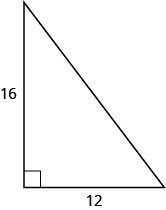Exercise $$\PageIndex{27}$$25

Exercise $$\PageIndex{28}$$In the following exercises, use the Pythagorean Theorem to find the length of the leg. Round to the nearest tenth, if necessary.

Exercise $$\PageIndex{29}$$8

Exercise $$\PageIndex{30}$$Exercise $$\PageIndex{31}$$12

Exercise $$\PageIndex{32}$$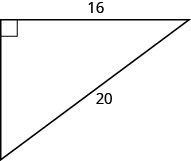Exercise $$\PageIndex{33}$$10.2

Exercise $$\PageIndex{34}$$Exercise $$\PageIndex{35}$$9.8

Exercise $$\PageIndex{36}$$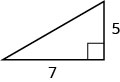In the following exercises, solve using the Pythagorean Theorem. Approximate to the nearest tenth, if necessary.

Exercise $$\PageIndex{37}$$

A 13-foot string of lights will be attached to the top of a 12-foot pole for a holiday display, as shown below. How far from the base of the pole should the end of the string of lights be anchored?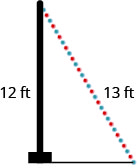5 feet

Exercise $$\PageIndex{38}$$

Pam wants to put a banner across her garage door, as shown below, to congratulate her son for his college graduation. The garage door is 12 feet high and 16 feet wide. How long should the banner be to fit the garage door?Exercise $$\PageIndex{39}$$

Chi is planning to put a path of paving stones through her flower garden, as shown below. The flower garden is a square with side 10 feet. What will the length of the path be?14.1 feet

Exercise $$\PageIndex{40}$$

Brian borrowed a 20 foot extension ladder to use when he paints his house. If he sets the base of the ladder 6 feet from the house, as shown below, how far up will the top of the ladder reach?Solve Applications Using Rectangle Properties

In the following exercises, solve using rectangle properties.

Exercise $$\PageIndex{41}$$

The length of a rectangle is 85 feet and the width is 45 feet. What is the perimeter?

260 feet

Exercise $$\PageIndex{42}$$

The length of a rectangle is 26 inches and the width is 58 inches. What is the perimeter?

Exercise $$\PageIndex{43}$$

A rectangular room is 15 feet wide by 14 feet long. What is its perimeter?

58 feet

Exercise $$\PageIndex{44}$$

A driveway is in the shape of a rectangle 20 feet wide by 35 feet long. What is its perimeter?

Exercise $$\PageIndex{45}$$

The area of a rectangle is 414 square meters. The length is 18 meters. What is the width?

23 meters

Exercise $$\PageIndex{46}$$

The area of a rectangle is 782 square centimeters. The width is 17 centimeters. What is the length?

Exercise $$\PageIndex{47}$$

The width of a rectangular window is 24 inches. The area is 624 square inches. What is the length?

26 inches

Exercise $$\PageIndex{48}$$

The length of a rectangular poster is 28 inches. The area is 1316 square inches. What is the width?

Exercise $$\PageIndex{49}$$

Find the length of a rectangle with perimeter 124 and width 38.

24

Exercise $$\PageIndex{50}$$

Find the width of a rectangle with perimeter 92 and length 19.

Exercise $$\PageIndex{51}$$

Find the width of a rectangle with perimeter 16.2 and length 3.2.

4.9

Exercise $$\PageIndex{52}$$

Find the length of a rectangle with perimeter 20.2 and width 7.8.

Exercise $$\PageIndex{53}$$

The length of a rectangle is nine inches more than the width. The perimeter is 46 inches. Find the length and the width.

16 in., 7 in.

Exercise $$\PageIndex{54}$$

The width of a rectangle is eight inches more than the length. The perimeter is 52 inches. Find the length and the width.

Exercise $$\PageIndex{55}$$

The perimeter of a rectangle is 58 meters. The width of the rectangle is five meters less than the length. Find the length and the width of the rectangle.

17 m, 12 m

Exercise $$\PageIndex{56}$$

The perimeter of a rectangle is 62 feet. The width is seven feet less than the length. Find the length and the width.

Exercise $$\PageIndex{57}$$

The width of the rectangle is 0.7 meters less than the length. The perimeter of a rectangle is 52.6 meters. Find the dimensions of the rectangle.

13.5 m length, 12.8 m width

Exercise $$\PageIndex{58}$$

The length of the rectangle is 1.1 meters less than the width. The perimeter of a rectangle is 49.4 meters. Find the dimensions of the rectangle.

Exercise $$\PageIndex{59}$$

The perimeter of a rectangle is 150 feet. The length of the rectangle is twice the width. Find the length and width of the rectangle.

50 ft., 25 ft.

Exercise $$\PageIndex{60}$$

The length of a rectangle is three times the width. The perimeter of the rectangle is 72 feet. Find the length and width of the rectangle.

Exercise $$\PageIndex{61}$$

The length of a rectangle is three meters less than twice the width. The perimeter of the rectangle is 36 meters. Find the dimensions of the rectangle.

7 m width, 11 m length

Exercise $$\PageIndex{62}$$

The length of a rectangle is five inches more than twice the width. The perimeter is 34 inches. Find the length and width.

Exercise $$\PageIndex{63}$$

The perimeter of a rectangular field is 560 yards. The length is 40 yards more than the width. Find the length and width of the field.

160 yd., 120 yd.

Exercise $$\PageIndex{64}$$

The perimeter of a rectangular atrium is 160 feet. The length is 16 feet more than the width. Find the length and width of the atrium.

Exercise $$\PageIndex{65}$$

A rectangular parking lot has perimeter 250 feet. The length is five feet more than twice the width. Find the length and width of the parking lot.

85 ft., 40 ft.

Exercise $$\PageIndex{66}$$

A rectangular rug has perimeter 240 inches. The length is 12 inches more than twice the width. Find the length and width of the rug.

Everyday Math

Exercise $$\PageIndex{67}$$

Christa wants to put a fence around her triangular flowerbed. The sides of the flowerbed are six feet, eight feet and 10 feet. How many feet of fencing will she need to enclose her flowerbed?

24 feet

Exercise $$\PageIndex{68}$$

Jose just removed the children’s playset from his back yard to make room for a rectangular garden. He wants to put a fence around the garden to keep out the dog. He has a 50 foot roll of fence in his garage that he plans to use. To fit in the backyard, the width of the garden must be 10 feet. How long can he make the other length?

Writing Exercises

Exercise $$\PageIndex{69}$$

If you need to put tile on your kitchen floor, do you need to know the perimeter or the area of the kitchen? Explain your reasoning.

Exercise $$\PageIndex{70}$$

If you need to put a fence around your backyard, do you need to know the perimeter or the area of the backyard? Explain your reasoning.

Exercise $$\PageIndex{71}$$

Look at the two figures below.1. Which figure looks like it has the larger area?
2. Which looks like it has the larger perimeter?
3. Now calculate the area and perimeter of each figure.
4. Which has the larger area?
5. Which has the larger perimeter?
4. The areas are the same.
5. The 2x8 rectangle has a larger perimeter than the 4x4 square.

Exercise $$\PageIndex{72}$$

Write a geometry word problem that relates to your life experience, then solve it and explain all your steps.

Self Check

ⓐ After completing the exercises, use this checklist to evaluate your mastery of the objectives of this section.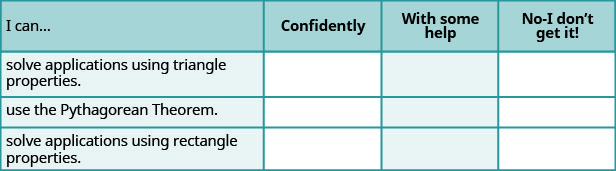ⓑ What does this checklist tell you about your mastery of this section? What steps will you take to improve?